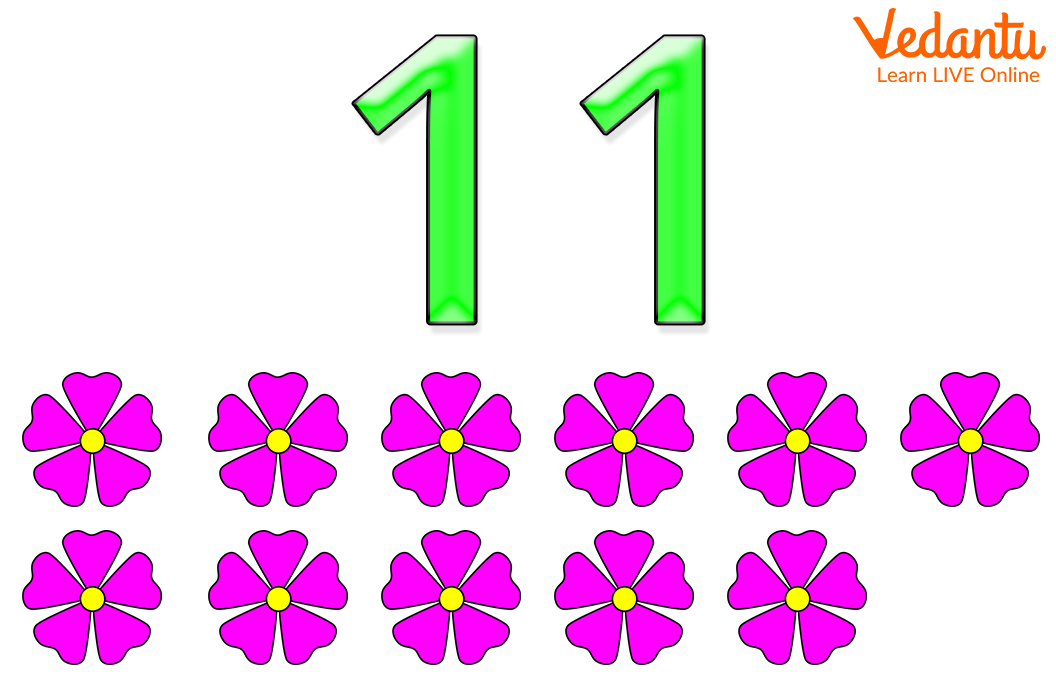Courses
Courses for Kids
Free study material
Free LIVE classes
More

# Learn with Fun about Number Eleven with Examples## What is an Eleven?

In mathematics, 11 is a number. The number 11 represents a value or quantity that is greater than 10. This is a whole number that lies between 10 and 12. 11 is called by the number name “eleven”. Kids can learn counting easily when each number is introduced to them through examples. When children are familiar with numbers from 1 to 10 then the number 11 can be introduced to them. Let’s read the article and know about number eleven in detail.Number Eleven

## Examples of Eleven

A few examples of eleven are given below. When you count them you will find out that they are 11 in number. There are 11 flowers, 11 candies and 11 dogs. These are greater than 10 and less than 12.Eleven FlowersExamples of Eleven: 11 Candies and 11 Dogs

## Let's Sing the Song of Eleven!

The following song will help kids to learn about eleven fast. Let’s sing together.

Eleven little rabbits,

Dug a hole in the ground

To store eleven carrots from the farm

With hard work and mind!

## Tips for Parents!

• Parents can ask their kids to do activities that will help them to learn to count. They can ask their child to count or observe things around them that are 11 in number.

• One interesting activity that your child can do is to note down the pattern of multiples of 11.

• We should not just hand out worksheets to kids to learn and memorise numbers.

• We should discuss various facts related to a number. For example, for number eleven, we can give examples of the number of players in a cricket, soccer, American football team, etc.

## Do You know?

• The smallest two-digit prime number is 11.

• 11 is an odd number.

## Conclusion

Kids are always eager to learn and when learning is incorporated with fun, the concepts are grabbed very easily at an early stage. In this article, the number 11 is explained through examples so that kids can remember the number 11.

Last updated date: 29th Sep 2023
Total views: 128.4k
Views today: 1.28k

## FAQs on Learn with Fun about Number Eleven with Examples

1. How do you explain number 11 to your child?

It is obvious that when we are teaching or introducing the number 11 to our child, he or she must be aware of numbers from 1 to 10. We can teach them through examples of 11 things and put 11 things in front of them and ask them to count and then tell them that the number that comes after 10 is 11 and that the value of this number is greater than 10. We should always do small activities with kids to teach them. It makes learning easy and fun for them.

2. Is number 11 an even number or an odd number?

The numbers that are divisible by number 2 without remainders are called even numbers. Even numbers end in 0, 2, 4, 6, or 8. Odd numbers are not evenly divisible by number 2 and they end in 1, 3, 5, 7, or 9. Hence, the number 11 is an odd number.